Chapter 6

As we know, the electromagnetic field has the properties of energy, momentum and angular momentum. The electromagnetic field momentum density is: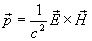(6.1)

Based upon the electromagnetic field momentum, and then we can define the field angular momentum density as follows: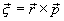(6.2)

Thus: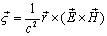(6.3)

Then integrating equation (6.3), we can get the field angular momentum: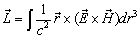(6.4)

Combine the equation (4.2) and (5.2) into equation (6.3),

Thus:(6.5)

For the cylindrical coordinate (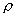,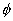, z), which has the follows relationship with the spherical coordinate (r,,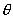):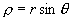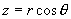We can separate the angular momentum density into the z component andcomponent,

The z component of angular momentum density is: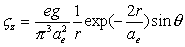(6.6)

Thecomponent of angular momentum density is: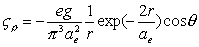(6.7)

As we know the volume element is: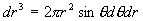For thecomponent of angular momentum density, because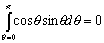(6.8)

Thus we can get thecomponent of angular momentum: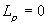For the z component of electron angular momentum, we have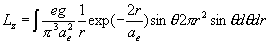(6.9)

Thus: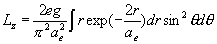(6.10)(6.11)

Therefore: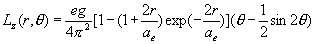(6.12)

The equation (6.12) is the angular momentum distribution equation of an electron.

When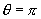, the z component of angular momentum is: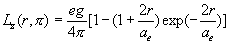(6.13)

The equation (6.13) is the angular momentum within the sphere of radius r of an electron.

The angular momentum distribution is a kind of cumulative gamma distribution in mathematics [B]

When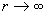thus: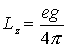(6.14)

The above angular momentum is the electron’s electromagnetic field angular momentum in total.

What is the electron spin? As we know, the electron spin is the electron intrinsic angular momentum. Let us make an assumption that electron spin is the electromagnetic field angular momentum, which also means that the electron spin is of purely electromagnetic origin.

Thus: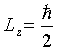(6.15)

Combine (6.14) and (6.15), thus: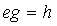(6.16)

From equation (6.16), we found out that the multiple of electric charge unit ‘e’ and magnetic charge unit ‘g’ equal the Planck’s constant ‘h.

Then, we can also calculate the ratio of magnetic charge ‘g’ and electric charge ‘e’ as follows: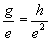(6.17)

Thus: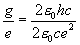(6.18)

So: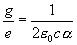(6.19)

Thus: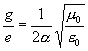(6.20)

As we know, the vacuum impedance is: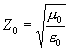(6.21)

So we can see the ratio of magnetic charge unit ‘g’ and electric charge unit ‘e’ has the unit of impedance.

Reference

Douglas Singleton, Jerzy Dryzek

Phys. Rev. B, 62, 13070 (2000)

D. Singleton
Am. J. Phys. 66, 697 (1998)

J. S. Trefil

Am. J. Phys. 51, 1113 (1983)

N. Gauthier

Am. J. Phys. 70, 1034 (2002)

Jerzy Dryzek , Douglas Singleton

Am. J. Phys. 67, 930 (1999)

Electromagnetic momentum density and the Poynting vector in static fields
Francis S. Johnson, Bruce L. Cragin, and R. Richard Hodges

Am. J. Phys. 62, 33 (1994)

David J. Griffiths

Am. J. Phys. 60, 979 (1992)

Peter H. Ceperley

Am. J. Phys. 60, 938 (1992)

V. Hnizdo

Am. J. Phys. 60, 242 (1992)

Antonio S. de Castro

Am. J. Phys. 59, 180 (1991)

K. R. Brownstein

Am. J. Phys. 57, 420 (1989)

Jack Higbie

Am. J. Phys. 56, 378 (1988)

H. S. T. Driver

Am. J. Phys. 55, 755 (1987)

Hans C. Ohanian

Am. J. Phys. 54, 500 (1986)

Robert H. Romer

Am. J. Phys. 53, 15 (1985)

A. C. Lawson

Am. J. Phys. 50, 946 (1982)

E. Corinaldesi

Am. J. Phys. 48, 83 (1980)

Ph. M. Kanarev

Journal of Theoretics Vol.4-4

Ph. M. Kanarev

APEIRON Vol. 7 Nr. 3-4, July-October, 2000